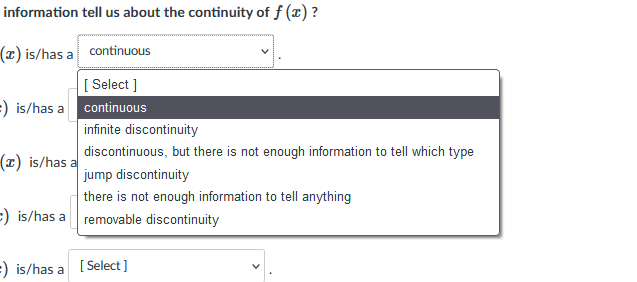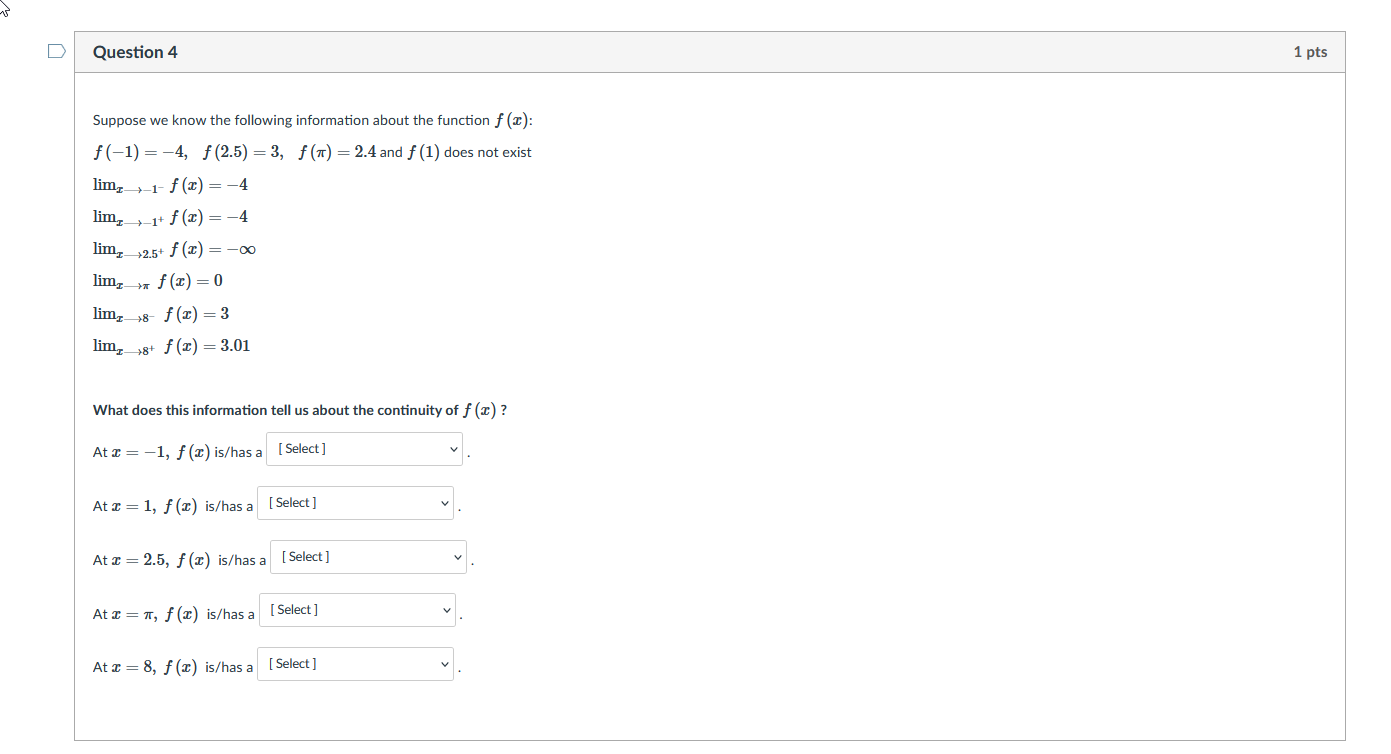# Question Solved1 AnswerOptions: information tell us about the continuity of f(x)? (2) is/has a continuous [ Select] ) is/has a continuous infinite discontinuity discontinuous, but there is not enough information to tell which type (2) is/has a jump discontinuity there is not enough information to tell anything -) is/has a removable discontinuity =) is/has a [Select] w Question 4 1 pts Suppose we know the following information about the function f (x): f(-1) = -4, f(2.5) = 3, f(1) = 2.4 and f(1) does not exist lim,_-1-f(x) = -4 lim,-1+ f(x) = -4 lim, 2.5+ f(x) = -0 lim, f() = 0 lim, 18. f(2)=3 lim,_18+ f2) = 3.01 What does this information tell us about the continuity of f(x)? At <= -1, f() is/has a Select] At x = 1, f() is/has a [Select] At I=2.5, f(c) is/has a [ Select ] At <=, f(x) is/has a [Select] At I=8, f(c) is/has a [ Select]Options:Transcribed Image Text: information tell us about the continuity of f(x)? (2) is/has a continuous [ Select] ) is/has a continuous infinite discontinuity discontinuous, but there is not enough information to tell which type (2) is/has a jump discontinuity there is not enough information to tell anything -) is/has a removable discontinuity =) is/has a [Select] w Question 4 1 pts Suppose we know the following information about the function f (x): f(-1) = -4, f(2.5) = 3, f(1) = 2.4 and f(1) does not exist lim,_-1-f(x) = -4 lim,-1+ f(x) = -4 lim, 2.5+ f(x) = -0 lim, f() = 0 lim, 18. f(2)=3 lim,_18+ f2) = 3.01 What does this information tell us about the continuity of f(x)? At <= -1, f() is/has a Select] At x = 1, f() is/has a [Select] At I=2.5, f(c) is/has a [ Select ] At <=, f(x) is/has a [Select] At I=8, f(c) is/has a [ Select]
More
Transcribed Image Text: information tell us about the continuity of f(x)? (2) is/has a continuous [ Select] ) is/has a continuous infinite discontinuity discontinuous, but there is not enough information to tell which type (2) is/has a jump discontinuity there is not enough information to tell anything -) is/has a removable discontinuity =) is/has a [Select] w Question 4 1 pts Suppose we know the following information about the function f (x): f(-1) = -4, f(2.5) = 3, f(1) = 2.4 and f(1) does not exist lim,_-1-f(x) = -4 lim,-1+ f(x) = -4 lim, 2.5+ f(x) = -0 lim, f() = 0 lim, 18. f(2)=3 lim,_18+ f2) = 3.01 What does this information tell us about the continuity of f(x)? At <= -1, f() is/has a Select] At x = 1, f() is/has a [Select] At I=2.5, f(c) is/has a [ Select ] At <=, f(x) is/has a [Select] At I=8, f(c) is/has a [ Select]